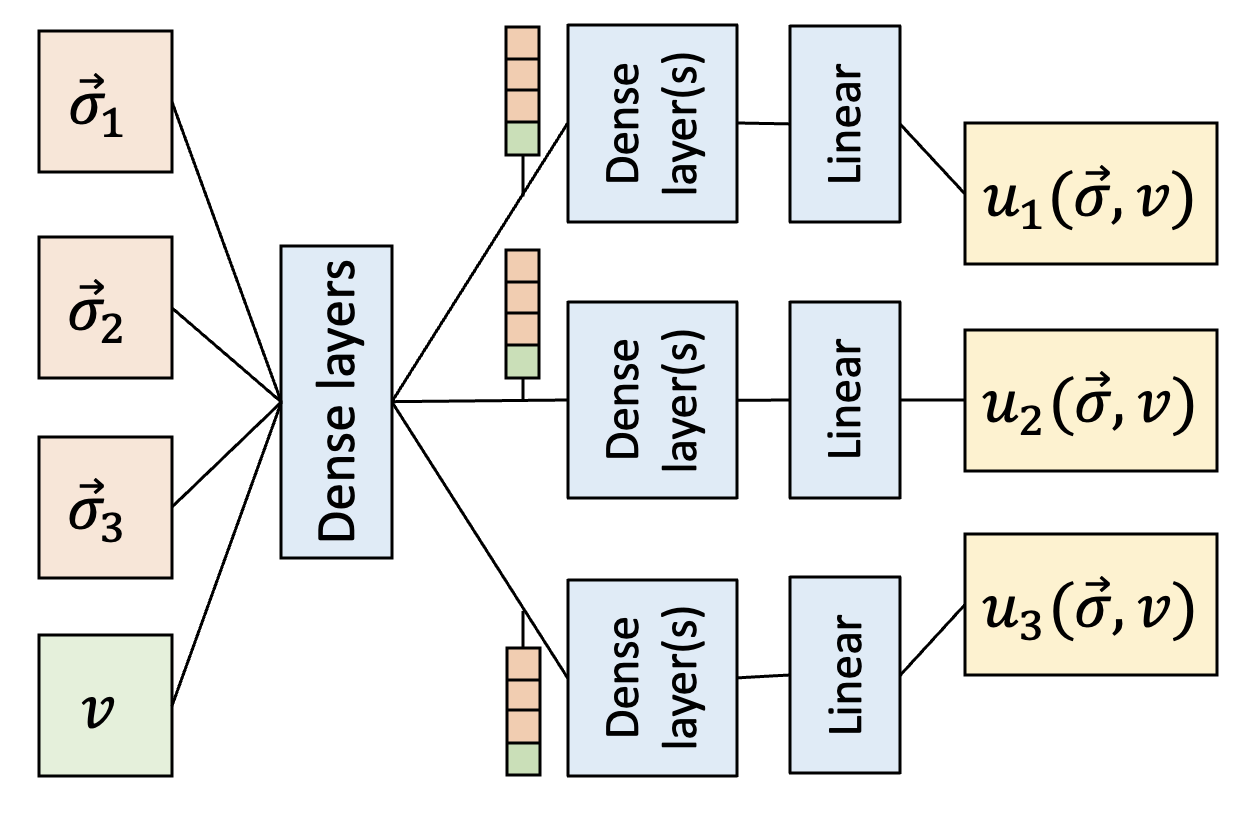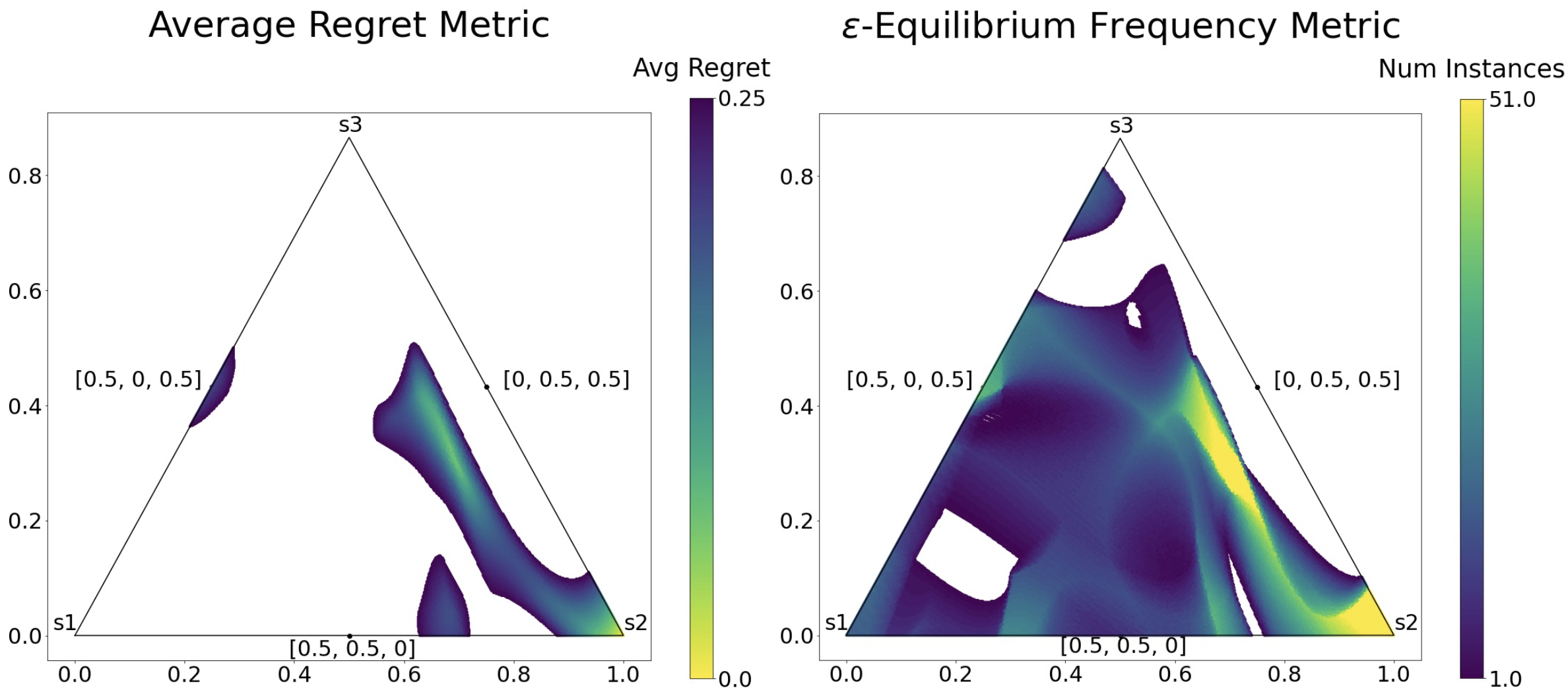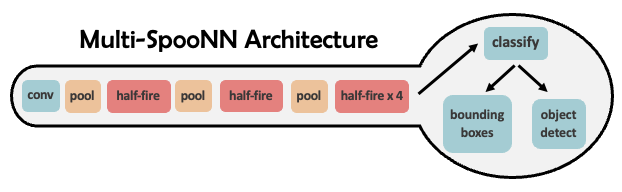## Research### Learning Parameterized Families of Games

To understand the impact of parameters in strategic environments, typical game-theoretic analysis involves selecting a small set of representative values and constructing and analyzing separate game models for each value. We introduce a novel technique to learn a single model representing a family of closely related games that differ in the number of symmetric players or other ordinal environment parameters. Prior work trains a multi-headed neural network to output mixed-strategy deviation payoffs, which can be used to compute symmetric epsilon-Nash equilibria. We extend this work by making environment parameters into input dimensions of the regressor, enabling a single model to learn patterns which generalize across the parameter space. For both continuous and discrete parameters, our results show that these generalized models outperform existing approaches, achieving better accuracy with roughly half as much data. This technique makes thorough analysis of the parameter space more tractable, and promotes analyses that capture relationships between parameters and incentives.

AAMAS-23: PDF### Variable-Player Learning for Simulation-Based Games

We introduce a novel technique that uses a multi-headed neural network to analyze symmetric simulation-based games with a large, variable number of players, where the number of participants falls in a specified range. Our work extends prior work by converting what would otherwise be an extrinsic hyperparameter of a normal-form game into a dimension of the machine learning model. This allows a single model to learn patterns that generalize across a range of player values, which in turn enables types of analyses that compare or show robustness over the range of player values. We use this learned model to approximate robust equilibria, strategy profiles that represent reasonable predictions of behavior over a wide range of player counts. Finally, we present several possible measures of equilibrium robustenss, and compare their results in experiments on synthetic games.